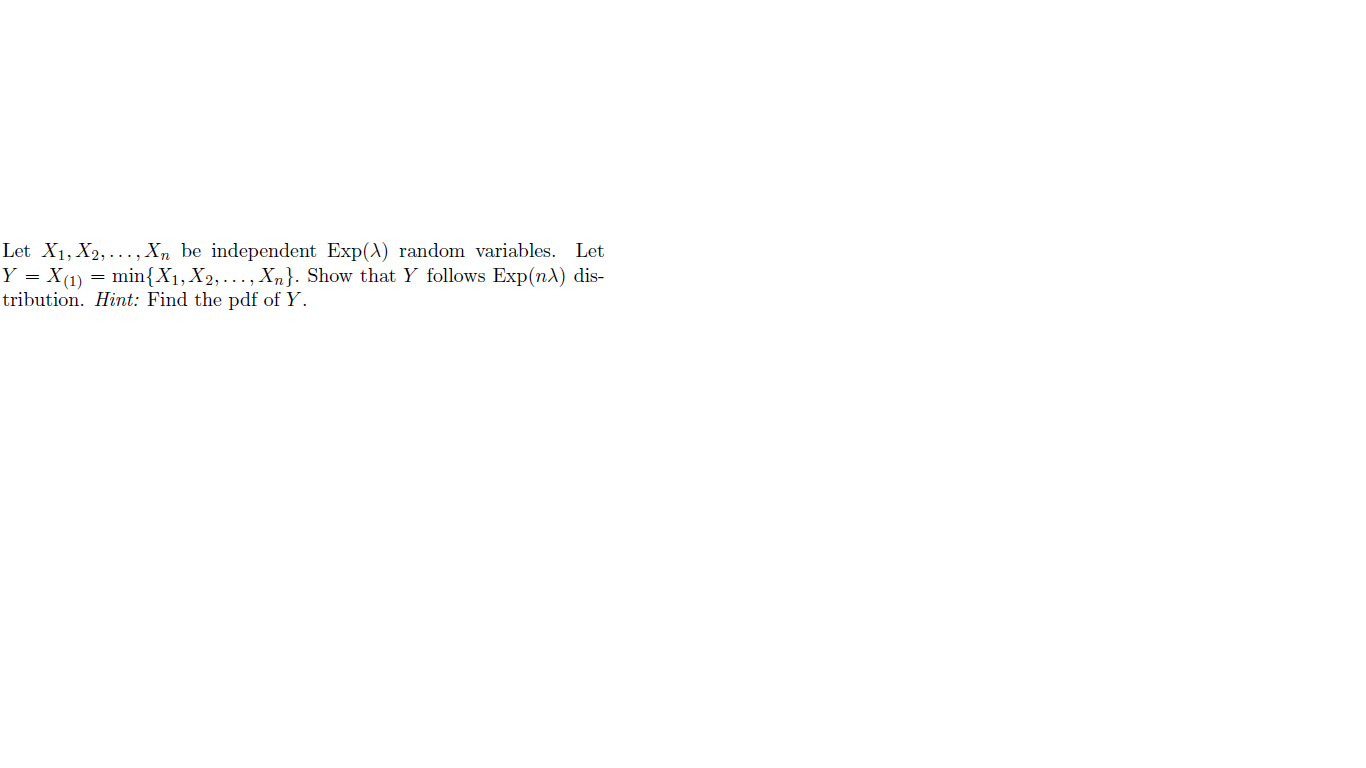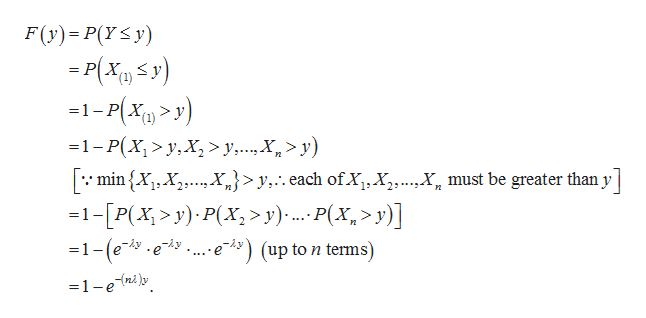# Let X1, X2,... , Xn be independent Exp(A) random variables. LetY = X(1)min{X1, X2, ... , Xn}. Show that Y follows Exp(nA) dis-tribution. Hint: Find the pdf of Y

Question
10 viewshelp_outlineImage TranscriptioncloseLet X1, X2,... , Xn be independent Exp(A) random variables. Let Y = X(1)min{X1, X2, ... , Xn}. Show that Y follows Exp(nA) dis- tribution. Hint: Find the pdf of Y fullscreen
check_circle

Step 1

Note:

Hey there! Thank you for the question. Although the hint in the question suggests finding the pdf of Y to solve the problem, we have used a different method- the cdf- to solve it. Both ways are correct and possible to use here.

Step 2

Introduction:

Consider an exponential distribution with probability density function (pdf):

f (x) = λ e – λx; x > 0; λ > 0.

The cumulative distribution function (cdf) for this distribution is:

P (Xx) = F (x) = 1 – e – λx; x > 0.

Therefore, the tail function is given as:

P (X > x) = e – λx; x > 0.

Step 3

Calculation:

It is given that Y = X(1) = min {X1, X2, …, Xn}.

Note that, as X1, X2, …, Xn are independent, their joint cdf fill be the product of their individual cdf’s; as a result, their joint tail function would also be the product of their ind...help_outlineImage TranscriptioncloseF(y) P(YS y) P(X(y) 1-P(Xy) -1-P(X1>y,X > y,...X >y) min X, XX}>y,..each ofX,X,Y must be greater than y -1-[P(x>y) P(X,> y)... P(Xx>y)] 1-(ee-t9 2 2 e9(up to n ternms) =1-ey fullscreen

### Want to see the full answer?

See Solution

#### Want to see this answer and more?

Solutions are written by subject experts who are available 24/7. Questions are typically answered within 1 hour.*

See Solution
*Response times may vary by subject and question.
Tagged in

### Random Variables